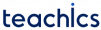# Hall effect Transducers

Hall effect transducers can measure the magnetic field strength. Direct measurement of magnetic field intensity is impracticable and hence, this kind of transducer uses the Hall effect for this purpose. Hall effect is the production of e.m.f or voltage difference across a current-carrying conductor in the presence of a magnetic field, which is perpendicular to both the current and magnetic field.

The magnitude of voltage depends on the strength of the magnetic field, current and properties of the conductor material used. A voltmeter can be used to measure the magnitude of the produced voltage.

The voltage developed due to Hall effect or the Hall Effect e.m.f is given asWhere,
KH is a constant of proportionality known as the Hall Effect Coefficient.
B is the magnetic field intensity.
I is the current through the conductor.
T is the thickness of the conductor.

• Long life.
• High-speed operation.

• May be affected by an external magnetic field.
• High temperature affects the sensitivity of the transducer.

### Applications

• Position sensing.
• Magnetometer.
• Automotive fuel level indicator.
• Encoded Switches.
Subscribe
Notify of